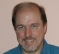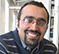## s_to_z (Pupalaikis)Started by 8 years ago10 replieslatest reply 8 years ago232 views
Paper: Bilinear Transformation Made Easy - http://documents.mx/documents/easybilinearpdf.html

I get the same output for Butterworth lp example there so, I suppose my implementation is OK.
Now, I'm trying to generate coefficients for de-emphasis filter by using it's time constants which are

p1 = 3180e-6; // 50.05
p2 = 75e-6; // 2.122k
z1 = 318e-6; // 500.5
z2 = 0.0; // not always zero

Now I'm stuck in what to do for those time constants before putting them into s_to_z() call? Which are the steps needed? Also, is that 'pre-warping' always needed to do?

[ - ]I have an old .m file for this kind of thing: deemphasis.m
[ - ]Thanks. That's a handy tool in many tasks. Hopefully it works in octave.

I need a solution for real time calculations so it does not give the answer for my query. I have an open source project on going where I'm trying to implement using C#/C++ the software which I made as prototype using Max/MSP:In prototype I used MZT but it does not give good results (at close Nyqvist) at low sample rates so in these C implementations I have to try with BLT, which is said to give better results...

Juha

[ - ]First, you turn the time constants into frequencies.

Second, you prewarp those frequencies (and hence pole and zero locations).

Third, you make numerator and denominator coefficients in the Laplace domain out of the pole and zero locations.

Fourth, if you want, you make a Laplace-domain transfer function.

Finally, you call s_to_z() with the numerator coefficients, and call it again with the denominator coefficients.

[ - ]Thanks. So far I'm not familiar with other but MZT so be patient...

1. done

2. done

3. how's this done?

4. do you mean exp(something) or H = {s^2-s*(z1+z2)+z1*z1}/{s^2-s*(p1+p2)+p1*p2} ?

5. yep

Juha

[ - ]1:

p1 = 3180e-6; // 50.05
p2 = 75e-6; // 2.122k
z1 = 318e-6; // 500.5
z2 = 0.0; // not always zero

Playing fast and loose with notation:

p1 = 1/(3180e-6 sec) = 314 radian/sec
p2 = 1/(75e-6 sec) = 13333 radian/sec

z1 = 1/(318e-6 sec) = 3145 radian/sec

2:

I have to assume a sampling rate here.  I'm going to use 50kHz, because it's audio-ish, and while nothing I know of samples at 50kHz, it's a nice round number.  So:

p1w = 2 * atan((314 rad/sec) / (100 kHz)) = 0.00628
p2w = 2 * atan((13333 rad/sec) / (100kHz)) = 0.26510

z1w = 2 * atan((3145 rad/sec) / (100kHz)) = 0.03144

(Note the use of units here -- this will get you out of so much trouble it's impossible to overlook.  Just remember that tan(any unit) is impossible, and go from there).

These didn't "warp" much, which is a hopeful sign.

3:

Numerator: s + z1w = s + 0.03144

Denominator: (s + p1w)(s + p2w) = (s + .00628)(s + 0.26510)
= s^2 + 0.27138s + 0.001664828

(Precision is important here if you're cranking it by hand: the behavior of the filter depends on the positions of the poles, which are much more sensitive to that final coefficient than they are to the middle one.  This is one of the joys of IIR filter implementation.)

(Precision is important here if you're doing it numerically, too: the amount that the trailing coefficient can be off is roughly the amount that the slowest (lowest frequency) pole can wander off target, squared.  So if you don't want the low-frequency pole to move by more than 10%, you need the s^0 coefficient to be accurate to about (0.006)^2, or about 0.000036.  Use doubles to do your calculations, and if you're using fixed-point to implement the filter pay particular attention to numerical effects.  Rick Lyon's "Understanding Digital Signal Processing" goes into this, as does my "Applied Control Theory for Embedded Systems".  Well, and just about any decent DSP book.)

4:

Numerator
H(z) = -----------
Denominator

s + 0.03144
= ----------------------------
s^2 + 0.27138s + 0.001664828

[ - ]Thank you very much for taking your time and explaining this process with an example. As my math/DSP skills are not very good, those equations found on papers looks soo 'cryptic'...

[ - ]Papers aren't for casual reading -- you generally have to sit down and study them, often with reference works, a notepad, a pencil, and a great big eraser.

[ - ]One more question.

Now when you use rad/sec units there in 1-4, does it mean it has to be same units all the way (i.e. in s_to_z() call as well)?

[ - ]To transform the Laplace domain to z domain, we can use the z=exp(j w Ts) mapping to map the imaginary axis to the unit circle where Ts=1/fs denotes the sampling time.

To simplify the transform people use bi-linear which is an approximation (z=exp(sT)=(1+sT/2)/(1-sT/2)). To use this transform we already know the H(s) and Ts.

To obtain the H(z), assuming the poles and zeros above,

H(s)=(s-z1)(s-z2)/((s-p1)(s-p2))

s=2/T(z-1)/(z+1)=>

(s-z1) ---->    A=(2/T(z-1)/(z+1)-z1),  (s-z2) ---->    B=(2/T(z-1)/(z+1)-z2)

(s-p1) ---->    C=(2/T(z-1)/(z+1)-p1),  (s-p2) ---->    D=(2/T(z-1)/(z+1)-p2)

Then you can get the final H(z)=H(s=2/T(z-1)/(z+1))=(A*B/(C*D)).

This will give you the filter numerator and denominator.

To check the frequency response just you can do:

Hz(exp(jw))=Hs(j2/T tan(wT/2)) (Hz is digital, Hs is analog))which is the frequency wrapping function.

Hope this helps.

[ - ]Thanks!

As I mentioned already I'm not good with math/DSP so, I would try your suggestion as well but ... there are few unknown variables in those equations which ones I can only quess. What are j, w, sT and plain T?

In MZT implementation which I'm using in my prototype, everything seems so simple when coded (my example filter):

fs = 44100.0;

p1 = exp(-1.0/(fs*3180e-6))

p2 = exp(-1.0/(fs*75e-6))

z1 = exp(-1.0/(fs*318e-6))

z2 = exp(-1.0/(fs*0.0))

and then get the num/den

d = 1.0;
d = -(p1+p2);
d = p1*p2;
n = 1.0;
n = -(z1+z2);
n = z1*z2;

... but as MZT has aliasing issues near Nyqvist frequency ...